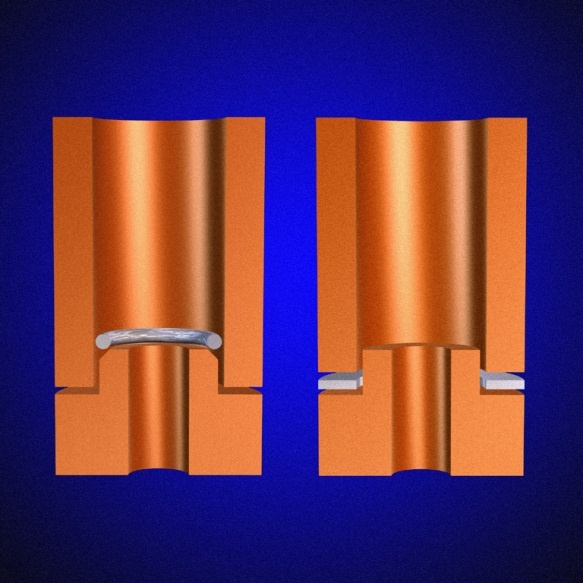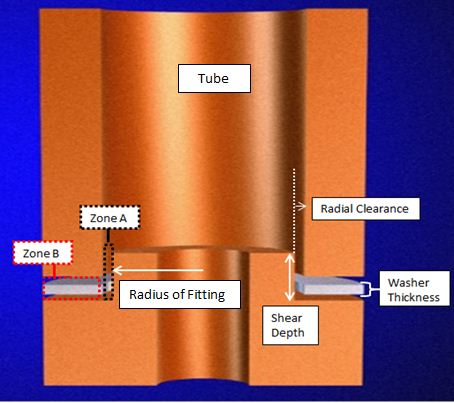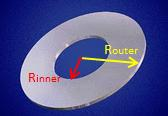Ring vs. Washer
Both rings and washers are preform shapes used for brazing. For smaller parts with relatively small joint volume and lap area, either a washer or ring may be used (Figure 1).Both washers and rings can be circular in shape; however, their cross sections will always differ in shape. A washer has rectangular cross section, whereas a ring has a circular cross section.

Calculating Joint Volume
In order to know how much alloy you need, you must first calculate the volume of the joint you are filling. You must ensure that the braze alloy can fill the shear depth, regardless of where the components fit within their tolerances. Thus, it is important to measure for worst-case scenario.

Figure 1: Ring preform application on the left and washer preform application on the right.

For your joint calculation, the inner-dimension value of the tube should be the largest-possible value within tolerance, and the outer dimension value of the fitting should be the smallest-possible value within tolerance. This will give you the largest clearance and hence, your calculated volume will be sufficient for the worst-case scenario. An approximation of the joint volume follows:Zone A = SD * 2πRFitting * Cm

Where:

SD = Shear depth

2πRFitting = Circumference of the Fitting

Cm = Maximum Radial Clearance = (IDmaxTube - ODminFitting)/2

Figure 2:
Joint calculation

The calculation above is for determining the volume between the fitting and the tube vertically (Zone A); however, this is only a fraction of the total joint volume. You must also account for the space between the two parts in the horizontal orientation (Zone B):

Zone B = (πRouter^2- πRInner^2)*h

Where:

πRouter^2 = Outer area (ODTube/2)

πRouter^2 = Inner area (ODFitting/2)

h = Height (The Space between bottom of tube and the top of the fitting where washer will be placed. Note: the height of final braze melt is not the same as the washer, which normally is between .002"-.005".)

Your joint volume will be the sum of both zones:

Joint Volume = Zone A + Zone B

The washer volume for this application will equal your calculated joint volume plus a 15% safety factor to account for fillet and variation in surface roughness.

Calculating Washer Volume
Next, use the washer volume to calculate the thickness of material you would need for the washer. The inner diameter (ID) of the washer is equal to the outer diameter (OD) of the fitting it goes around, and the OD of the washer will be equal to the OD of the outer-most fitting wall.

Washer Volume = (πRouter^2- πRinner^2)*ThicknessWhere:

πRouter^2 = Outer area

πRinner^2 = Inner area

Figure 3: Washer

An example of a washer calculation follows:

SD = .500in
OD fitting = .254in ± .002in
ID tube = .260in ± .002in
OD tube = .750in ± .002in
H = .002in

The dimensions that would make the joint clearance largest are used in the equation. Looking at the sample data, the IDmax of the tube is .262in and the ODmin of the fitting is .252in. These dimensions yield the largest joint clearance. The maximum radial clearance used would be half of the IDmax of the tube minus the ODmin of the fitting. In calculating the circumference of the fitting, the radius of the fitting used is .127in without taking the tolerance into account. The calculation for joint volume is shown below.

SD = .500in
Cm = (.262in -.252in)/2 = .005in

Zone A = SD * 2πRFitting * Cm
Zone A = .500in * 2π(.127in) * .005in
Zone A = .002 in^3

Zone B = (πRouter^2- πRInner^2)*h
Zone B = (π ((.750+.002)/2)^2 - π((.260-.002)/2)^2)*.002
Zone B = 7.8 X 10^-4 in^3

Joint volume = .002 in^3 + 7.8 X 10^-4 in^3
Joint volume
= .00278 in^3

(1.15)Joint Volume = Washer Volume
Washer Volume = (πRouter^2- πRInner^2)*Thickness 0-.002)/2)^2)*Thickness
.003197 in^3 = .39in^2 * Thickness
.0032 in^3/.39in^2 = Thickness
.0082in = Thickness

Conclusion
The success of a braze joint is dependent on the proper design of a preform. To ensure a proper braze joint, the volume of alloy should match or exceed the volume of the joint to be brazed. Using a calculated washer preform will ensure consistent application and hence a successful braze operation.